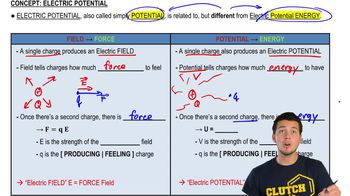Start typing, then use the up and down arrows to select an option from the list.
5:28 minutes
Problem 23a
Textbook Question

# At a certain distance from a point charge, the poten-tial and electric-field magnitude due to that charge are 4.98 V and 16.2 V/m, respectively. (Take V = 0 at infinity.) (a) What is the distance to the point charge?Verified Solution
This video solution was recommended by our tutors as helpful for the problem above.
96views